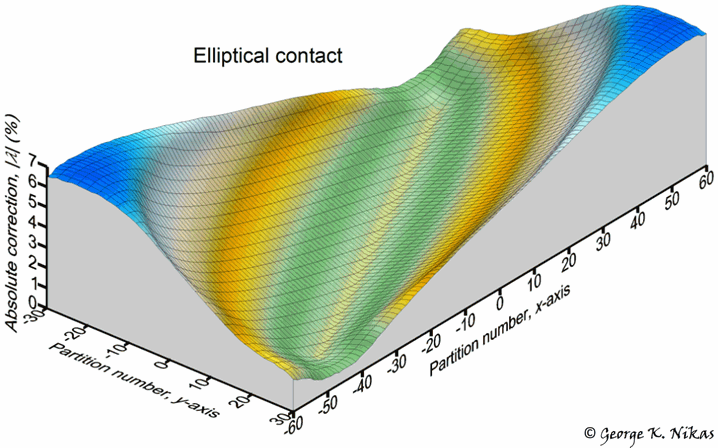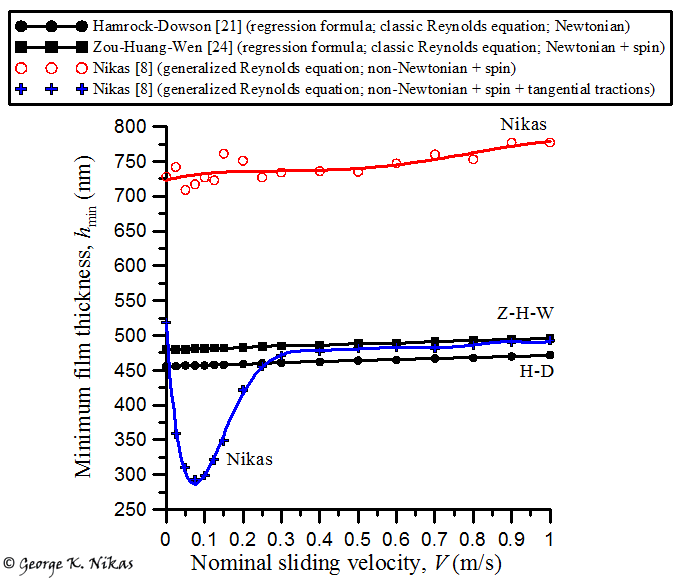Paper: Miscalculation of film thickness, friction and contact efficiency by ignoring tangential tractions in elastohydrodynamic contacts.

Author: George K. Nikas

Published in: Tribology International, 2017, 110, 252-263

Errata: Two lines below Eq. (A9), in the viscosity equation, the right square bracket should be positioned to the right of the "-1". Moreover, in Eqs. (A13), τL should be raised to the Λ power. These are only misprints in the published paper.

Abstract

Traction in elastohydrodynamic contacts causes normal surface displacements, affecting the lubricant film thickness. This is ignored in the vast majority of published theoretical studies and film-thickness regression formulae. This article analytically formulates traction effects on normal displacements, establishing the most contributing factors and the associated displacement error when tractions are ignored, which can exceed 20%. Numerical tests on a rolling-sliding-spinning elastohydrodynamic elliptical contact and comparison with well-known published formulae show that the central film thickness is unaffected by the traction factor simplification but the minimum film thickness is occasionally significantly overestimated. Traction and contact efficiency are also in error but usually by less than 1%. Correspondingly, wear may also be miscalculated, particularly in contacts with sliding and/or spinning motion.

Highlights

• Exact formulation of normal surface displacements in concentrated contacts.
• Numerical error calculation on normal displacements when tractions are ignored.
• Study of numerical errors in rolling-sliding-spinning, elastohydrodynamic, elliptical contacts.
• Analysis of numerical errors on the central and minimum film thickness.
• Analysis of numerical errors on traction coefficient and contact efficiency.
• Comparison with published results and regression formulae.

Some figures and details from this work

The thickness of the liquid film separating two elastohydrodynamically lubricated surfaces is the sum of the geometrical separation of the unloaded surfaces simply touching each other and the normal surface displacements caused by the pressure developed in squeezing the film. When there is any sliding motion between the co-operating surfaces, there is friction or traction. Traction is thus the shear stress on the co-operating surfaces caused by shearing layers of the lubricating film in the process of sliding. Friction (tangential traction) is in turn causing normal surface displacements according to well established equations in the context of contact mechanics. However, normal surface displacements from such tractive loading are simply ignored in almost all published studies to date dealing with elastohydrodynamic numerical analysis, which is equivalent to treating an elastohydrodynamic contact as frictionless! Depending on the direction of local traction in a contact, the film thickness may be increased or decreased. Whilst increasing the local film thickness is generally good for reducing or avoiding wear, reducing the local film thickness can prove bad or even catastrophic. It is therefore vital to account for traction-induced normal surface displacements to correctly evaluate the performance of a contact be it in terms of friction and wear.

In the author's article, the effect of tangential tractions on normal surface displacements is generally developed through analytical (algebraic) equations. Using some simple contact scenarios for demonstration, namely a uniformly pressurized contact obeying Amontons' friction laws (i.e., friction is proportional to pressure), it is shown that normal surface displacements caused by friction can exceed 20% of those caused by pressure alone in some cases. It is proved that the relative error in displacement calculations is a specific function of the local traction coefficient, the Poisson's ratio, the modulus of elasticity and the ratio of the principal dimensions of the contact, factors which are subsequently discussed in the article through various examples. Figure 1 below shows an example of said error for a greasy elliptical contact between steel surfaces with Coulomb friction coefficient equal to 0.2 and Poisson's ratio equal to 0.3, which is uniformly pressurized and also sheared in both principal directions x and y.Fig. 1. Example of the normal displacement error by ignoring tangential tractions. A greasy elliptical contact between uniformly pressurized steel surfaces, which are also sheared in both principal directions x and y. In this example, the Coulomb friction coefficient and the Poisson's ratio are equal to 0.2 and 0.3, respectively.

After discussing these effects via the proposed analytical formulation in the article, the study continues with the more important case of a real elastohydrodynamic contact with combined rolling, sliding and spinning motion, which refers to a commercial traction drive. In that scenario, the numerical problem is too complex to analyse algebraically and requires a numerical solution. Indeed this is done by utilizing a generalized Reynolds equation for non-Newtonian lubrication of isothermal, smooth contacts developed and validated in an earlier publication of the author. Results on the central and minimum film thickness are obtained parametrically for a wide range of rolling velocities, sliding velocities and ellipticity ratios. These are subsequently compared with published regression formulae such as that by Hamrock and Dowson, which are widely accepted in the literature. The comparisons reveal that, whilst the central film thickness is unaffected by the traction factor simplification, the minimum film thickness is significantly overestimated at low rolling velocity, low sliding velocity and/or low ellipticity ratio. An example is shown in Fig. 2 below.Fig. 2. Comparison of the minimum film thickness by the author's numerical results and that calculated by well-known regression formulae. Note the fundamental differences among the models, not only in terms of rheology and spin effects but also in terms of the fundamental Reynolds equation used in the analyses (generalized with allowance for viscosity variation across the film plus a local shear stress limitation versus classical, which lacks such features). The red line by the author neglects tangential tractions, whilst the blue line accounts for them. The reduction of the minimum film thickness for 0 < V < 0.25 m/s by account of tangential tractions (blue line) is mathematically proved in the article by linking it to the local sliding velocity variation.

In addition to film thickness calculations for the aforementioned traction-drive contact, the article discusses parametric results on the overall traction coefficient and the contact efficiency. Those also reveal some significant occasional errors but in most cases, the relative error remains lower than 1%, which is quite low except when dealing with high powered transmissions, as for example, 300 kW. In conclusion, the traction factor simplification found in existing numerical codes and incorporated in published regression formulae is an approximation that can have critical consequences in cases or sliding and/or spinning contacts by overestimating the minimum film thickness and, correspondingly,  underestimating the wear rate or risk of surface damage in the long term.

Homepage of Dr Nikas